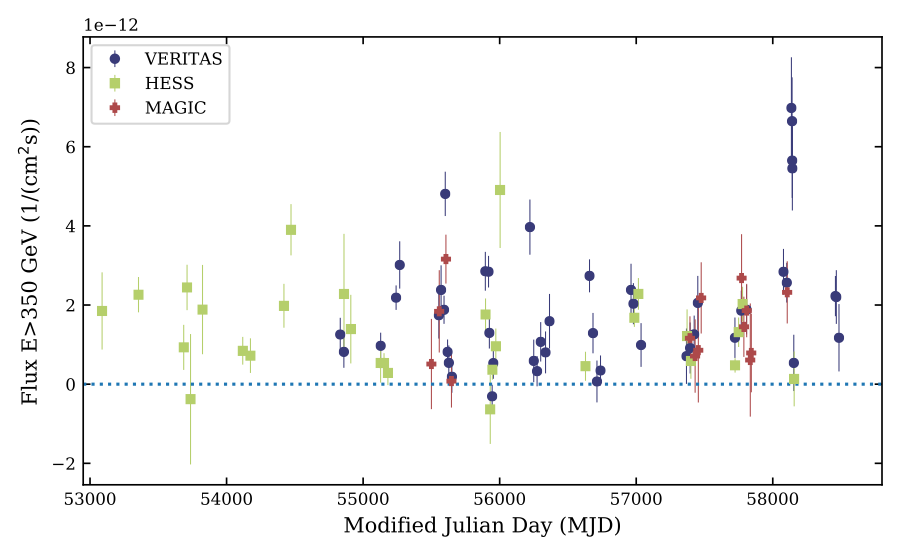Gamma-ray light curve for HESS 0632+057; see Figure 2 (below) for more details.

#### Contacts: Gernot Maier

A gamma-ray binary consists of a compact object, like a neutron star or a black hole, orbiting a massive star, with periodic release of large amounts of non-thermal emission at energies >1 MeV. There are only a few of these unusual objects known and new observations are key to better understanding how particles are accelerated to TeV energies in the environment of these objects.

The results of more than 15 years of observations of the gamma-ray binary HESS J063057 are presented in this publication. Data taken with the atmospheric Cherenkov telescopes H.E.S.S., MAGIC, and VERITAS at energies above 350 GeV were used together with observations at X-ray energies obtained with Swift -XRT, Chandra, XMM
-Newton, NuSTAR, and Suzaku. Some of these observations were accompanied by measurements of the Halpha emission line. This wealth of data allows for the first time to determine the dependency of the gamma-ray emission over the complete orbital phase, and discuss correlations of the emission at different wavelength in detail. A completely new result comes from the analysis of data of four orbital cycles with dense observational coverage. The VERITAS observations reveal short timescale variability, with flux-decay timescales of less than 20 days at very high energies. In addition, flux variations observed in the full data set obtained with all three instruments over the time scale of several years indicate orbit-to-orbit variability.

FITS files: N/A

Selected figures from paper (click to get full size image):

Figure 2:  Top: Long-term observations of HESS J0632+057 in X-rays (0.3–10 keV) with Swift-XRT, XMM-Newton, Suzaku, Chandra and NuSTAR. Bottom: Long-term observations of HESS J0632+057 in gamma rays (>350 GeV) with H.E.S.S., MAGIC, and VERITAS. Vertical lines indicate 68% statistical uncertainties; note that these are smaller than the marker size for all X-ray instruments except Swift-XRT.
Figure 3: X-ray (0.3–10 keV; top) and gamma-ray (>350 GeV; bottom) light curves as a function of the orbital phase, assuming an orbital period of 317.3 days
(MJD0=54857.0; Bongiorno et al. (2011)). Vertical error bars indicate statistical uncertainties; note that these are smaller than the marker size for all X-ray instruments except for Swift-XRT. Fluxes are averaged over time intervals indicated by the horizontal lines (for most flux points these are smaller than the marker size).

Figure 5: Top: Contemporaneous gamma-ray (>350 GeV) vs X-ray (0.3–10 keV) integral fluxes. In total, 59 measurements were selected with X-ray measurements taking place during the period of gamma-ray observations. The dashed line and shaded area indicate the results of a linear fit to the data and the one sigma error range, respectively. Bottom: Discrete cross-correlation function (DCF) between gamma-ray and X-ray data assuming a bin width for the time lag Δτ= 5 days. The dashed lines indicate the 2, 3, and 4  confidence levels (from bottom to top) derived from 100,000 toy Monte Carlo light curves. Only correlation coefficients with time lags consistent with zero are significant.

Figure 9: Detailed light curves for four orbits with deep X-ray and gamma-ray coverage in gamma rays (H.E.S.S., MAGIC, VERITAS, >350 GeV; left axis) and X-rays (NuSTAR, Swift-XRT, 0.3-10 keV; right axis). Orbits are numbered following the start of the gamma-ray observations (phase zero of the Orbit 1 is at MJD 52953). The orbits 9 and 17 (upper two panels) represent high states of the source while the orbits 10 and 16 (lower two panels) represent low states. Vertical lines indicate statistical uncertainties. Fluxes are averaged over time intervals indicated by the horizontal lines (for most flux points smaller than the marker size). An orbital period of 317.3 days is assumed.